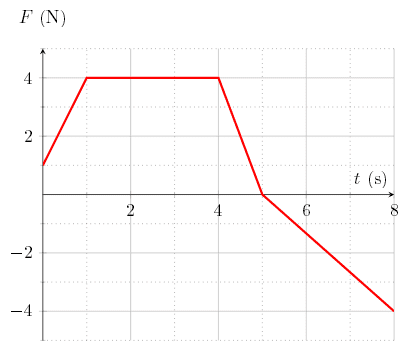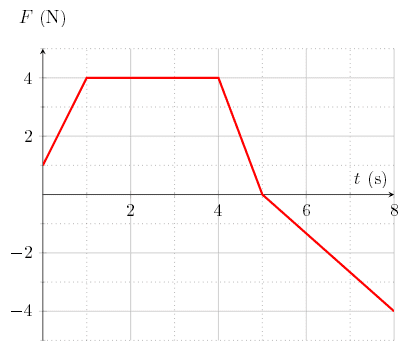# Classical mechanics: Force versus time graph

## Homework StatementA particle of mass m=4.0 kg is moving along the x-axis. The particle is being acted upon by a variable single force F⃗ , directed along the x-axis. At t=0 s, the particle is moving at v_0=−3 m/s.

What is the first time t>0 when the particle comes to a stop momentarily?

## The Attempt at a Solution

because I know the initial velocity ,I know the initial momentum and I know the final momentum too(as the particle stops temporarily) but the force is variable otherwise I would have equated change in momentum to the impulse imparted.and found the time t.

what should I do?

gneill
Mentor
What does area under a Force vs Time curve represent?

What does area under a Force vs Time curve represent?

it represents impulse.

##mv_f-mv_i=J##
##0-m(-3)=∫F. dt##

cnh1995
Homework Helper
Gold Member
it represents impulse.

##mv_f-mv_i=J##
##0-m(-3)=∫F. dt##
So you'll have to calculate the value of t at which the area of the curve becomes 12.

Last edited:
So you'll have to calculate the value of t at which the area of the curve becomes 12.

how will I do it? I don't have the force as a fn of time.

cnh1995
Homework Helper
Gold Member
I don't have the force as a fn of time.
You do.First you need to formulate F as a function of time between various intervals.

you need to formulate F as a function of time between various intervals.

you mean from 0 to 1 sec its 2.5
from 1 to 4 its 12

cnh1995
Homework Helper
Gold Member
Indeed it is. That's the final answer.
you mean from 0 to 1 sec its 2.5
from 1 to 4 its 12
No. From t=0 to t=1, what is F(t) and so on..

cnh1995
Homework Helper
Gold Member
Indeed it is. That's the final answer.

No. From t=0 to t=1, what is F(t) and so on..
Ok right, if you are talking in terms of areas directly..

if you are talking in terms of areas directly..

area under the f/t curve is all I know what else are you saying.also I am fairly new to calculus

cnh1995
Homework Helper
Gold Member
you mean from 0 to 1 sec its 2.5
from 1 to 4 its 12
Ok. So, from 0 to 4, the area is 14.5. So, t must be between 1 to 4. Agree? Because total area is 12 and out of that, 2.5 is already in between 0 to 1.

Ok. So, from 0 to 4, the area is 14.5. So, t must be between 1 to 4. Agree?

yes totally because of the areas.

cnh1995
Homework Helper
Gold Member
yes totally because of the areas.
So, from t=1 to t=4, you need to find at what value of t will the area be 9.5(i.e. 12-2.5). Can you do it?

cnh1995
Homework Helper
Gold Member
yes totally because of the areas.
You know its a rectangle from t=1 to t=4. You know the height. I believe the answer is in plain sight. Good luck..

•REVIANNA
•